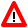MAXPREC - Maximum Number of Digits after Decimal Point

This Natural profile parameter is used to control Natural's compiler. This option determines the maximum number of digits after the decimal point that the Natural compiler generates for results of arithmetic operations.

Possible settings Default setting 7,…,29 The value denotes the maximum number of digits after the decimal point that the Natural compiler generates for results of arithmetic operations. The default value 7 provides upwards compatibility for existing applications. If such applications are cataloged with MAXPREC=7, they will deliver the same results as before. Objects cataloged with a Natural version that did not support the MAXPREC option are executed as if MAXPREC=7 had been set. If higher precision is desired for intermediate results, the value should be increased. The setting of MAXPREC does not limit the number of digits after the decimal point that can be specified for user defined fields and constants. However, the precision of such fields and constants influences the precision of results of arithmetic operations. This makes it possible to benefit from enhanced precision in selected computations without having the need to set the compiler option MAXPREC to a value that unintentionally affects other computations. So even if MAXPREC=7 is in effect, the following example program can be cataloged and executed: DEFINE DATA LOCAL 1 P (P1.15) END-DEFINE P := P + 0.1234567890123456 END See also Precision of Results of Arithmetic Operations in the Programming Guide. 7 yes yes OPTIONS MAXPREC Option of system command COMPOPTWarning:Changing the value of the MAXPREC option that is being used to catalog a Natural object may lead to different results, even if the object source has not been changed. See example below.

Example:

DEFINE DATA LOCAL
1 #R (P1.7)
END-DEFINE
#R := 1.0008 * 1.0008 * 1.0008
IF #R = 1.0024018 THEN ... ELSE ... END-IF

The value of #R after the computation and the execution of the IF statement depend on the setting of MAXPREC:

Setting of MAXPREC Effective at Compile Time Value of #R Executed Clause of IF Statement
MAXPREC=7 1.0024018 THEN clause
MAXPREC=12 1.0024019 ELSE clause